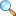# MTH633: Advanced Convex Analysis (Spring 2019)

Convex sets, convex hull, their properties, separation theorems, hyperplane, Best approximation theorem and its applications, Farkas and Gordan Theorems, Extreme points and Polyhedral. Convex functions, Basic Definitions, properties, various generalizations, differentiable convex functions, subgradient, characterization and applications in linear and nonlinear optimization, complementarity problems and its equivalent formulations.

• Assignment 02 | Download PDF |View Online• $\mathbb{R}$ and number sense
• $\mathbb{R}^n$ and subsets of $\mathbb{R}^n$
• Vector spaces including the concept of norm and inner product with examples.
• Euclidean space
• Concept of line with respect to vector in $\mathbb{R}$, $\mathbb{R}^2$ and in general $\mathbb{R}^n$.
• Affine set in $\mathbb{R}^n$
• Convex set in $\mathbb{R}^n$
• Half spaces
• Polyhedral
• Convex combination
• Convex hull
• Affince hull
• Cone and convex cone
• Affinely independent vectors
• Dimension of vector space, dimension of affine set and dimension of convex set.

Please click on View Online to see inside the PDF.

• Convex Analysis by R. Tyrrell Rockafellar.
• Convex Analysis By Jan van Tiel.
• Analysis: Convex Analysis and approximation theory‎by R. V. Gamkrelidze, Sergeĭ Mikhaĭlovich Nikolskiĭ.
• Abstract convex Analysis by Ivan Singer.
• atiq/sp19-mth633